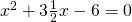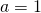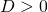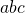# Solution assignment 16 Quadratic equations (factorizing)

### Assignment 16

Try to solve by factorization:### Solution

In this equation, but the coefficient ofis a fraction and factorizing cannot be applied easily. The equation has solutions since the discriminantand the-formula has to be applied, see Quadratic equations (abc-formula).

0